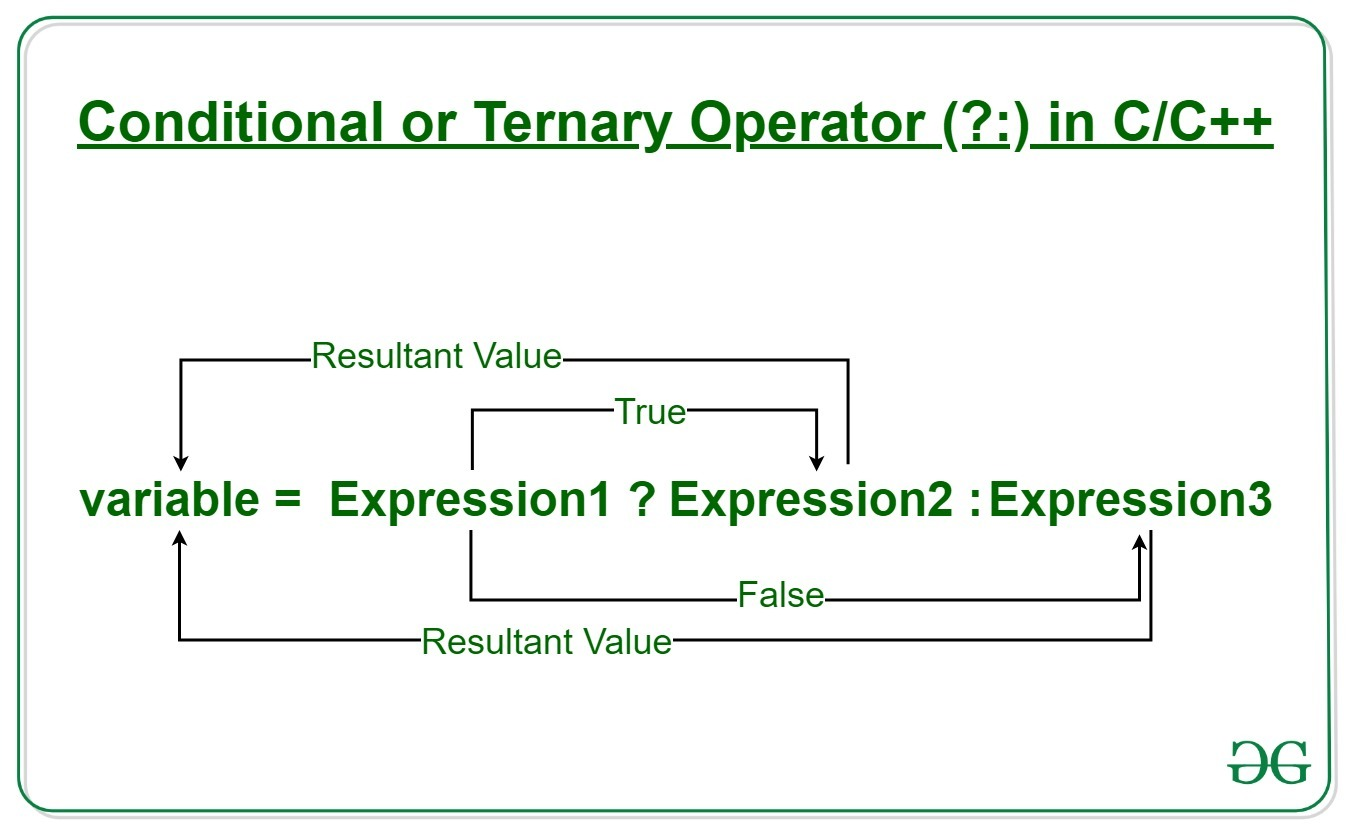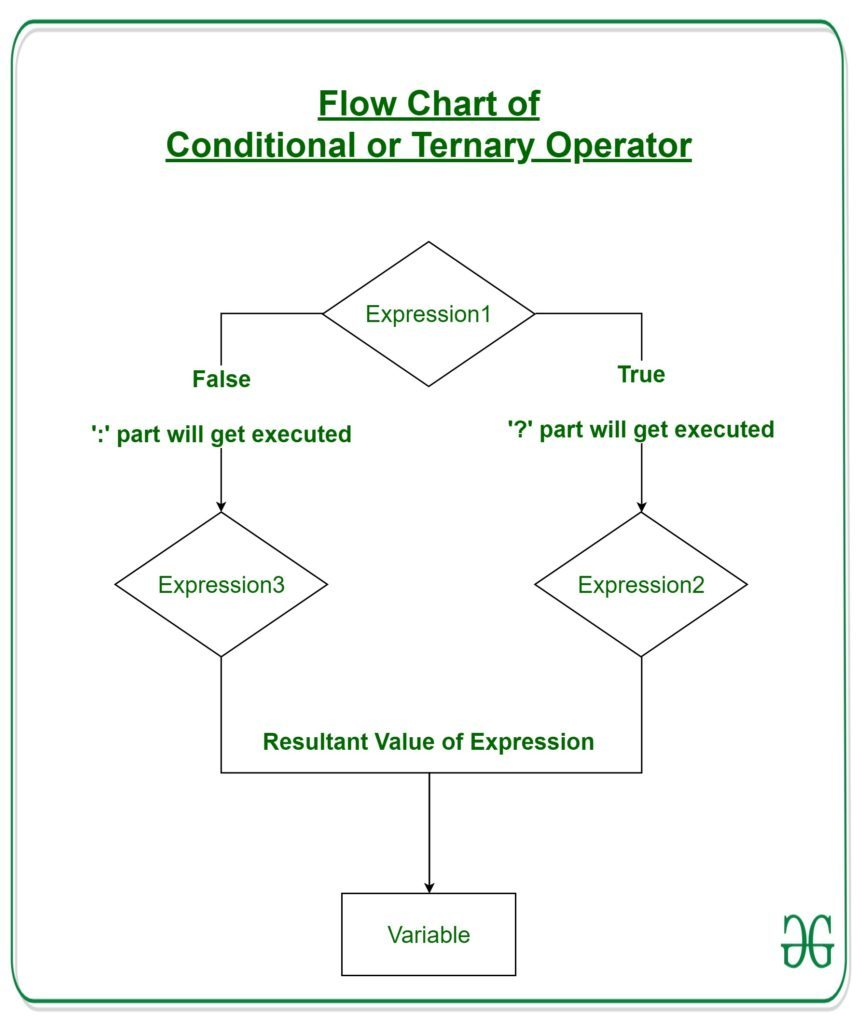Open in App
Not now

# Conditional or Ternary Operator (?:) in C/C++

• Difficulty Level : Easy
• Last Updated : 23 Jun, 2022

The conditional operator is kind of similar to the if-else statement as it does follow the same algorithm as of if-else statement but the conditional operator takes less space and helps to write the if-else statements in the shortest way possible.Syntax:
The conditional operator is of the form

`variable = Expression1 ? Expression2 : Expression3`

Or the syntax will also be in this form

`variable = (condition) ? Expression2 : Expression3`

Or syntax will also be in this form

`(condition) ? (variable = Expression2) : (variable = Expression3)`

It can be visualized into if-else statement as:

```if(Expression1)
{
variable = Expression2;
}
else
{
variable = Expression3;
}```

Since the Conditional Operator ‘?:’ takes three operands to work, hence they are also called ternary operators.
Working:
Here, Expression1 is the condition to be evaluated. If the condition(Expression1) is True then Expression2 will be executed and the result will be returned. Otherwise, if the condition(Expression1) is false then Expression3 will be executed and the result will be returned.Example 1: Program to Store the greatest of the two Number.

## C

 `// C program to find largest among two` `// numbers using ternary operator`   `#include `   `int` `main()` `{` `    ``int` `m = 5, n = 4;`   `    ``(m > n) ? ``printf``(``"m is greater than n that is %d > %d"``,` `                     ``m, n)` `            ``: ``printf``(``"n is greater than m that is %d > %d"``,` `                     ``n, m);`   `    ``return` `0;` `}`

## C++

 `// C++ program to find largest among two` `// numbers using ternary operator`   `#include ` `using` `namespace` `std;`   `int` `main()` `{` `    ``// variable declaration` `    ``int` `n1 = 5, n2 = 10, max;`   `    ``// Largest among n1 and n2` `    ``max = (n1 > n2) ? n1 : n2;`   `    ``// Print the largest number` `    ``cout << ``"Largest number between "` `<< n1 ` `                           ``<< ``" and "` `<< n2` `                           ``<< ``" is "` `<< max;`   `    ``return` `0;` `}`

Output

`m is greater than n that is 5 > 4`

Example 2: Program to check whether a year is leap year or not.

## C

 `// C program to check whether a year is leap year or not` `// using ternary operator`   `#include `   `int` `main()` `{` `    ``int` `yr = 1900;`   `    ``(yr%4==0) ? (yr%100!=0? ``printf``(``"The year %d is a leap year"``,yr)` `     ``: (yr%400==0 ? ``printf``(``"The year %d is a leap year"``,yr)` `         ``: ``printf``(``"The year %d is not a leap year"``,yr)))` `             ``: ``printf``(``"The year %d is not a leap year"``,yr);` `    ``return` `0;` `}`   `//This code is contributed by Susobhan AKhuli`

## C++

 `// C++ program to check whether a year is leap year or not` `// using ternary operator`   `#include ` `using` `namespace` `std;`   `int` `main()` `{` `    ``int` `yr = 1900;`   `    ``(yr%4==0) ? (yr%100!=0? cout<<``"The year "``<

Output

`The year 1900 is not a leap year`

My Personal Notes arrow_drop_up
Related Articles